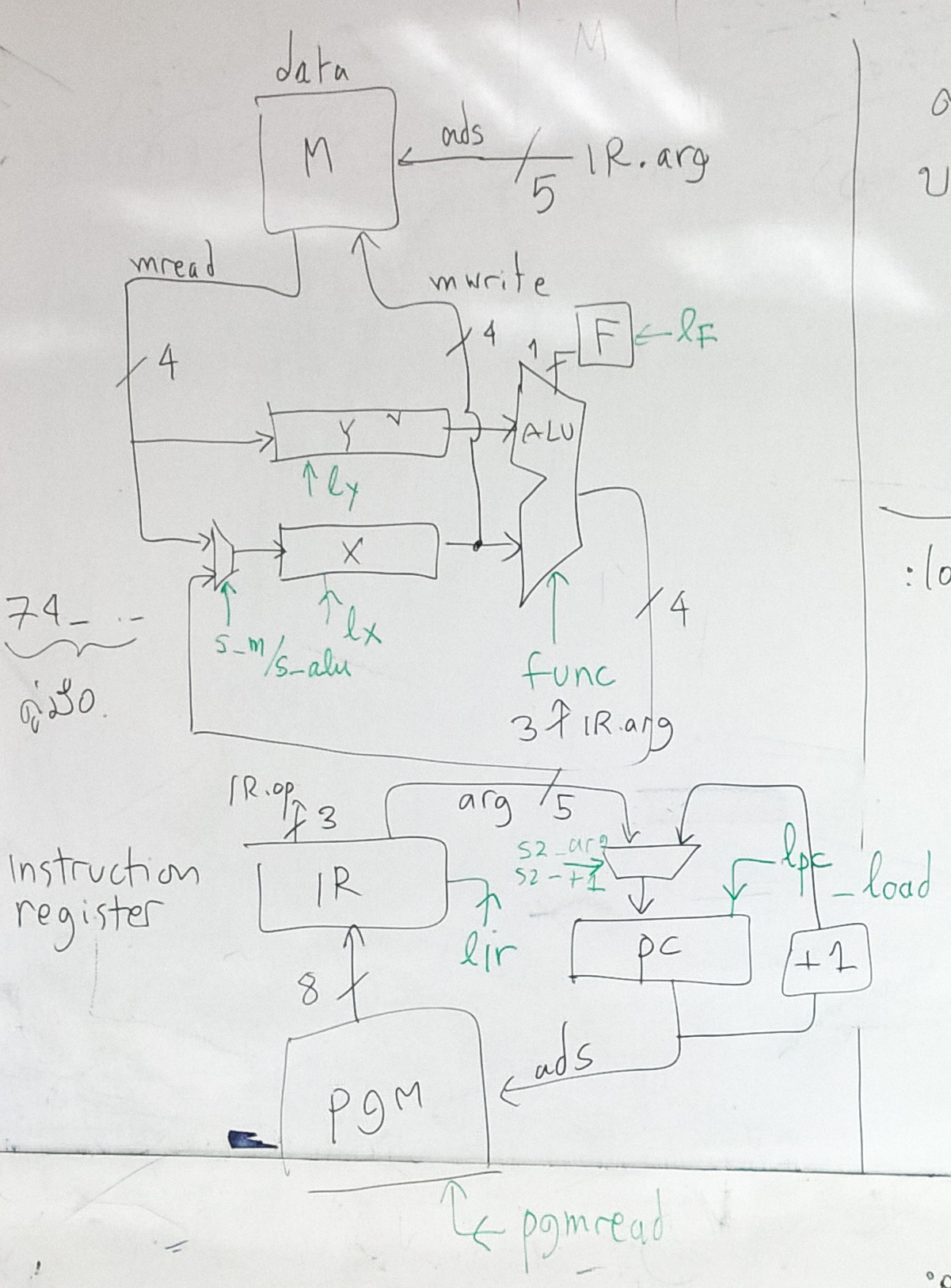# 4B processor

This is a 4-bit processor. Its goal is to be a processor that is small and simple enough to be implemented by basic logic elements without the need for a hardware description language.  The idea behind this design is to let students who learn elementary logic design to be able to implement this processor within one or two weeks.  The difficult part is the control logic.

The data width is 4 bits therefore it does not taking too much effort in implementing a data path (registers and ALU).  Students will work with the tools such as LogicWorks (or structural description).  They do not require to learn any hardware description language.  The testing of this processor can be done in a logic simulator (versus having an FPGA board). Therefore the learning curve to achieve a skill level that one can build a processor is very low.

To make a processor that is very small, its instruction set is severely limit. We decide to do with a fixed width instruction.  There is one format:

`op:3 arg:5`

The argument field is 5-bit therefore the maximum "addressable" memory space is 32.  The "program" and "data" are separate.  There are two 8-bit registers: X and Y and one bit flag.

### Instruction Set

code mnemonics
```1    ldx m     x = M[m] 2    ldy m     y = M[m] 3    stx m     M[m] = x 4    jmp ads   pc = ads 5    jT ads    if F then pc = ads 6    jF ads    if F == 0 then pc = ads 7    xop 0    stop      stop simulation ```
```xop 1    add       x = x + y 2    sub       x = x - y 3    inc       x = x + 1 4    dec       x = x - 1 5    eq        F = x == y 6    eqz       F = x == 0```<figure of 4B architecture>

### Example program

Add 1, 2, 3, 4 the result is 10.

`s = 0`
`i = 1`
`while i != 5`
`  s = s + 1`
`  i - i + 1`
`return s`

in assembly language of 4B

```:loop ldx i       ldy five       eq       jT exit       ldy s       add       stx s       ldx i       inc       stx i       jmp loop :exit stop```

last update 25 Sept 2017

Prabhas Chongstitvatana